Next: Constraints for the scalar-isovector Up: Continuity equations in the Previous: Constraints for zero-order terms

## Constraints for the scalar-isoscalar channel

For the standard CE in the scalar-isoscalar channel, Eq. (27), the constraints that we found from Eq. (48) are exactly the same as those defining the gauge-invariant functional up to N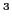LO [Carlsson et al.(2008)Carlsson, Dobaczewski, and Kortelainen]. This constitutes an explicit verification of the general result presented in Sec. 2.2.1. As in Ref. [Carlsson et al.(2008)Carlsson, Dobaczewski, and Kortelainen], we obtained two sets of independent constraints, one for the isoscalar coupling constants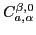and one for the isovector coupling constants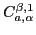. Within each isospin channel, the linear conditions for the scalar coupling constants,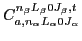, are disconnected from the linear conditions for the vector coupling constants,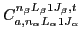.

Jacek Dobaczewski 2011-11-11